float Q_rsqrt( float number ) { long i; float x2, y; const float threehalfs = 1.5F;

x2 = number * 0.5F;
y  = number;
i  = * ( long * ) &y; // evil floating point bit level hacking i  = 0x5f3759df - ( i >> 1 ); // what the fuck?  y  = * ( float * ) &i;
y  = y * ( threehalfs - ( x2 * y * y ) ); // 1st iteration  // 2nd iteration, this can be removed // y  = y * ( threehalfs - ( x2 * y * y ) );  return y;
}

1x−−√1x

1x2+y2+z2−−−−−−−−−−√1x2+y2+z2

## 计算机的浮点数表示

• 第一段占 1bit。表示符号位。代称为 S（sign）。
• 第二段占 8bits。表示指数。代称为 E（Exponent）。
• 第三段占 23bits。表示尾数。代称为 M（Mantissa）。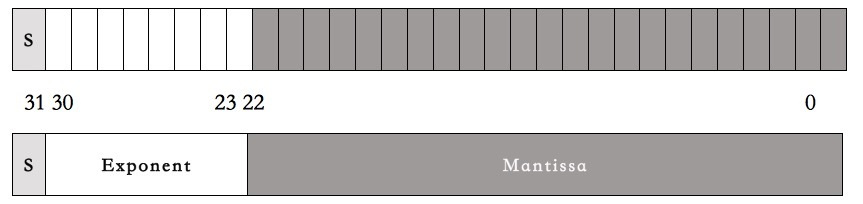(−1)S∗(1+M223)∗2(E−127)(−1)S∗(1+M223)∗2(E−127)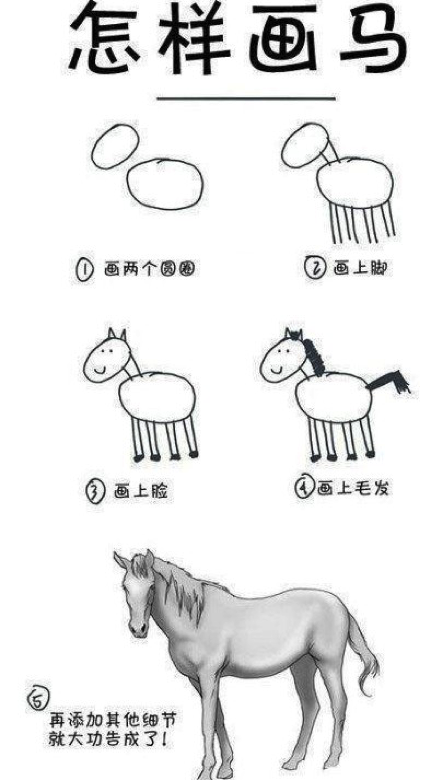• 第一段符号位。对于这一段，我相信应该没有人不能理解。

• 第二段指数位。什么叫指数？也就是说，对于任何数 x，其都可以找到一个 nn，使得 2n2n<=x<=2n+12n+1。比如：对于 3 来说，因为 2 < 3 < 4，所以 n=1。而浮点数的这个指数为了要表示 0.00x 的小数，所以需要有负数，这 8 个 bits 本来可以表示 0-255。为了表示负的，取值要放在 [-127,128] 这个区间中。这就是为什么我们在上面的公式中看到的 2(E−127)2(E−127) 这一项了。也就是说，n=E−127n=E−127，如果 n=1n=1，那么 EE 就是 128 了。

• 第三段尾数位。也就是小数位，但是这里叫偏移量可能好一些。这里的取值是从 [ 0 - 223223] 中。你可以认为，我们把一条线分成 223223 个线段，也就是 8388608 个线段。也就是说，把 2n2n 2n+12n+1 分成了 8388608 个线段。而存储的 M 值，就是从 2n2n 到 x 要经过多少个段。这要计算一下，2n2n 到 x 的长度占2n2n 2n+12n+1 长度的比例是多少。

• 是正数。所以，S = 0

• 2121 < 3.14 <2222。所以，n=1， n+127 = 128。所以，E=128。

• (3.14 - 2) / (4 - 2) = 0.57， 而 0.57∗223=4781506.560.57∗223=4781506.56，四舍五入，得到 M = 4781507。因为有四舍五入，所以，产生了浮点数据的精度问题。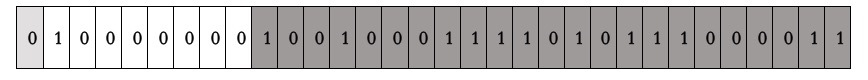(−1)0∗(1+4781507223)∗2(128−127)(−1)0∗(1+4781507223)∗2(128−127)
1∗(1+0.5700000524520874)∗21∗(1+0.5700000524520874)∗2
=3.1400001049041748046875=3.1400001049041748046875

• 是正数。所以，S = 0。

• 2−7<0.015<2−62−7<0.015<2−6 。所以，n=-7， n+127 = 120。所以，E=120。

• (0.015−2−7)/(2−6−2−7)(0.015−2−7)/(2−6−2−7) = 0.0071875/0.0078125=0.920.0071875/0.0078125=0.92。而 0.92∗223=7717519.360.92∗223=7717519.36，四舍五入，得到 M = 7717519。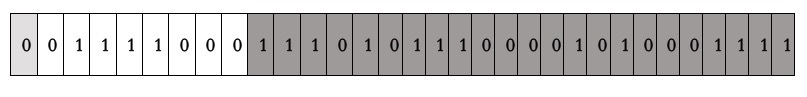• 120 的二进制是 01111000
• 7717519 的二进制是 11101011100001010001111

(−1)0∗(1+7717519223)∗2(120−127)(−1)0∗(1+7717519223)∗2(120−127)
(1+0.919999957084656)∗0.0078125(1+0.919999957084656)∗0.0078125
=0.014999999664724=0.014999999664724

int main() { float x = 3.14; float y = 0.015; return 0;
}

(lldb) frame variable
(float) x = 3.1400001 (float) y = 0.0149999997 (lldb) frame variable -f b
(float) x = 0b01000000010010001111010111000011 (float) y = 0b00111100011101011100001010001111 

## 简化浮点数公式

(−1)S∗(1+M223)∗2(E−127)(−1)S∗(1+M223)∗2(E−127)

(1+m)∗2e(1+m)∗2e

M+E∗223M+E∗223

7717519+120∗2237717519+120∗223
=1014350479=1014350479
=0X3C75C28F=0X3C75C28F

## 平方根倒数公式推导

y=1x−−√2=x−12y=1x2=x−12

log2(y)=−12log2(x)log2⁡(y)=−12log2⁡(x)

log2(1+my)+eylog2⁡(1+my)+ey
=−12(log2(1+mx)+ex)=−12(log2⁡(1+mx)+ex)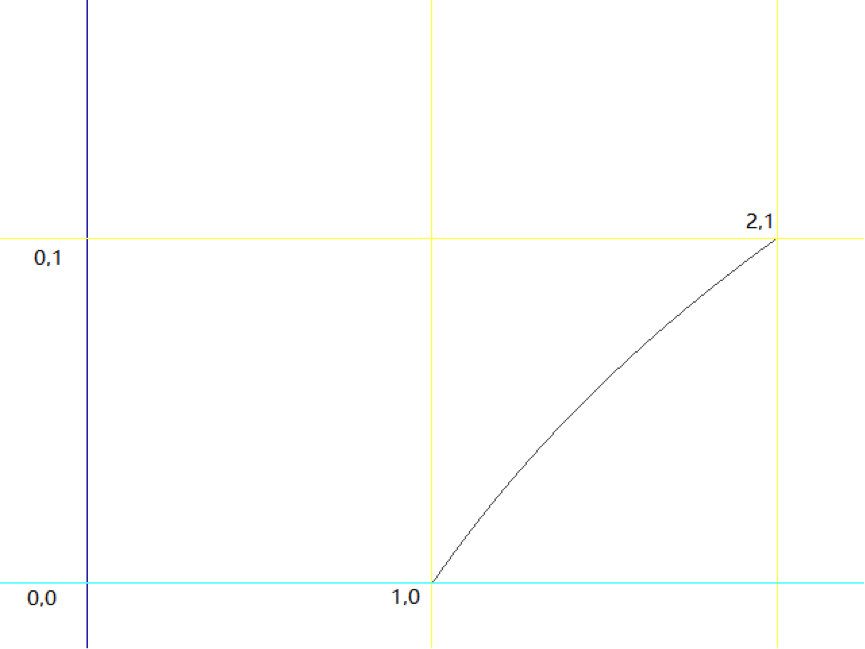log2(1+m)≈m+σlog2⁡(1+m)≈m+σ

my+σ+ey≈−12(mx+σ+ex)my+σ+ey≈−12(mx+σ+ex)

My223+σ+Ey−127My223+σ+Ey−127
≈−12(Mx223+σ+Ex−127)≈−12(Mx223+σ+Ex−127)

My223+Ey≈−12(Mx223+Ex)−32(σ−127)My223+Ey≈−12(Mx223+Ex)−32(σ−127)

My+Ey223My+Ey223
≈−12(Mx+Ex223)−32(σ−127)223≈−12(Mx+Ex223)−32(σ−127)223

My+Ey223≈R−12(Mx+Ex223)My+Ey223≈R−12(Mx+Ex223)

Iy≈R−12IxIy≈R−12Ix

## 代码分析

i  = * ( long * ) &y; // evil floating point bit level hacking

i  = 0x5f3759df - ( i >> 1 ); // what the fuck?

i = 0x5f3759df - ( i / 2 );

Iy≈R−12IxIy≈R−12Ix

x2 = number * 0.5F; y  = * ( float * ) &i;
y  = y * ( threehalfs - ( x2 * y * y ) );  // 1st iteration  // 2nd iteration, this can be removed
// y  = y * ( threehalfs - ( x2 * y * y ) );

Iy′=Iy(1.5−0.5xI2y)Iy′=Iy(1.5−0.5xIy2)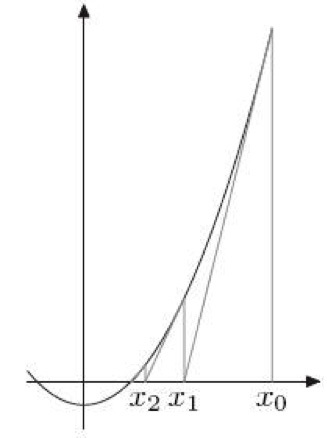xn+1=xn−f(xn)f′(xn)xn+1=xn−f(xn)f′(xn)

yn+1=yn−1y2n−x−2y3nyn+1=yn−1yn2−x−2yn3
=yn(3−xy2n)2=yn(1.5−0.5xy2n)=yn(3−xyn2)2=yn(1.5−0.5xyn2)

## 相关历史

Lomont 亦指出，64 位的 IEEE754 浮点数（即双精度类型）所对应的魔术数字是 0x5fe6ec85e7de30da。但后来的研究表明，代入 0x5fe6eb50c7aa19f9 的结果精确度更高（McEniry 得出的结果则是 0x5fe6eb50c7b537aa，精度介于两者之间）。